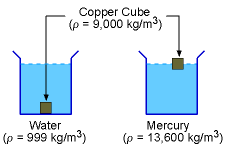Ch 1. Basics Multimedia Engineering Fluids MassDensity IdealGas Law Viscosity SurfaceTension VaporPressure
 Chapter 1. Basics 2. Fluid Statics 3. Kinematics 4. Laws (Integral) 5. Laws (Diff.) 6. Modeling/Similitude 7. Inviscid 8. Viscous 9. External Flow 10. Open-Channel Appendix Basic Math Units Basic Equations Water/Air Tables Sections Search eBooks Dynamics Fluids Math Mechanics Statics Thermodynamics Author(s): Chean Chin Ngo Kurt Gramoll ©Kurt GramollFLUID MECHANICS - THEORY

In this section, mass density will be introduced. The concept of the specific weight will also be presented.

Mass DensityMass DensityCopper Cube in Water and Mercury

The mass density (ρ) of a fluid is defined as the ratio of the mass of the fluid (m) to its volume (V). That is,

 ρ = m/V

The SI unit for the mass density is kg/m3 while the English unit is slugs/ft3.

For liquids, mass density is not a strong function of temperature and pressure. Hence, density is generally assumed to be independent of the temperature and pressure for liquids. On the other hand, for gases, density varies with both temperature and pressure. The relation between ρ, T and P for gases is given by the ideal gas law, which will be presented in a later section.

The density of different liquids has a wide range. For example, the density of water at 16oC is 999 kg/m3 while the density of mercury at 20oC is 13,550 kg/m3. In order for an object to float in a liquid, the density of the object must be less than that of the liquid. For example, a copper cube (ρ = 9,000 kg/m3) will sink in water but it will float on mercury.

 20°C (68°F) 1 atm Density, ρ (kg/m3) (slug/ft3) Water, pure 998 1.936 Water, sea 1,025 1.989 Ammonia 608 1.180 Benzene 881 1.709 Carbon Tetrachloride 1,590 3.085 Ethanol 789 1.531 Freon 12, liquid 1,327 2.575 Gasoline 680 1.319 Glycerin 1,260 2.445 Kerosene 804 1.560 Mercury 13,550 26.29 Methanol 791 1.535 SAE 10W Oil 870 1.688 SAE 30W Oil 891 1.729 SAE 50W Oil 902 1.750
For gases, a similar phenomenon can be observed. Consider the balloons, as shown in the figure. The density of helium inside the balloons is less than the surrounding air for a given temperature and pressure, hence the balloons rise in air. For more information on this subject, readers are referred to the discussion of buoyancy.

Specific Weight (or Weight Density)

The specific weight, γ, of a fluid is defined as

 γ = ρg

where g is the gravitational acceleration. Basically, the specific weight represents weight per unit volume. Sometimes it is also referred to as the weight density. The SI unit for the specific weight is N/m3 while the English unit is lb/ft3.

Specific Gravity

Specific gravity is simply the ratio of the specific weight of a given liquid to the specific weight of water at 4° C (γwater = 9.81 kN/m3 or 62.4 lb/ft3).

 SG = γ / γwater

This definition is helpful when comparing densities of different liquids.

Practice Homework and Test problems now available in the 'Eng Fluids' mobile app
Includes over 250 problems with complete detailed solutions.
Available now at the Google Play Store and Apple App Store.